# Search by Topic

#### Resources tagged with Equivalent fractions, decimals and percentages similar to A4 Fraction Subtraction:

Filter by: Content type:
Age range:
Challenge level:

### There are 10 results

Broad Topics > Fractions, Decimals, Percentages, Ratio and Proportion > Equivalent fractions, decimals and percentages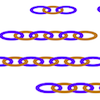##### Age 7 to 11 Challenge Level:

Can you find ways to make twenty-link chains from these smaller chains? This gives opportunities for different approaches.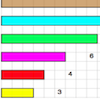### Fraction Lengths

##### Age 7 to 11 Challenge Level:

Can you find combinations of strips of paper which equal the length of the black strip? If the length of the black is 1, how could you write the sum of the strips?### The Greedy Algorithm

##### Age 11 to 14 Challenge Level:

The Egyptians expressed all fractions as the sum of different unit fractions. The Greedy Algorithm might provide us with an efficient way of doing this.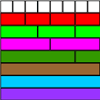### Fractional Wall

##### Age 7 to 11 Challenge Level:

Using the picture of the fraction wall, can you find equivalent fractions?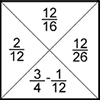### Fractions Jigsaw

##### Age 11 to 14 Challenge Level:

A jigsaw where pieces only go together if the fractions are equivalent.### Round and Round and Round

##### Age 11 to 14 Challenge Level:

Where will the point stop after it has turned through 30 000 degrees? I took out my calculator and typed 30 000 ÷ 360. How did this help?### Sept 03

##### Age 11 to 14 Challenge Level:

What is the last digit of the number 1 / 5^903 ?### Repetitiously

##### Age 11 to 14 Challenge Level:

The number 2.525252525252.... can be written as a fraction. What is the sum of the denominator and numerator?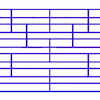### Tumbling Down

##### Age 7 to 11 Challenge Level:

Watch this animation. What do you see? Can you explain why this happens?### Fractions and Percentages Card Game

##### Age 11 to 16 Challenge Level:

Match the cards of the same value.